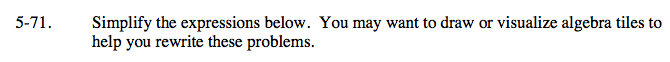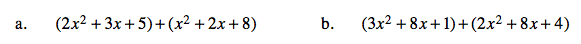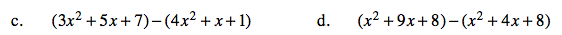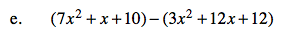Home > AC > Chapter 5 > Lesson 5.2.1 > Problem5-71

5-71.
1. Simplify the expressions below. You may want to draw or visualize algebra tiles to help you rewrite these problems. Homework Help ✎

1. (2x2 + 3x + 5) + (x2 + 2x + 8)

2. (3x2 + 8x + 1) + (2x2 + 8x + 4)

3. (3x2 + 5x + 7) − (4x2 + x + 1)

4. (x2 + 9x + 8) − (x2 + 4x + 8)

5. (7x2 + x + 10) − (3x2 + 12x + 12)Rearrange and put like terms together.
2x² + x² + 3x + 2x + 5 + 8

Combine like terms.

3x² + 5x + 13

Follow the steps in part (a).How does the negative outside the parentheses change what is inside of it?

Follow the steps in part (a).

x² + 4x + 6

Follow the steps in part (c).

5xFollow the steps in part (c).